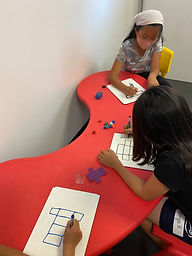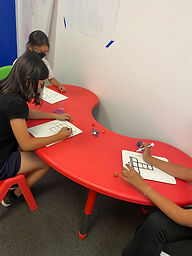## Ms. Briana

### Target 1​

###### Lesson Type:

Continuation

Measurment

:

Techniques and Formulas

Calculate the volume of rectangular prims.

###### 1:

Calculate volume from a geometric net.

6th

###### Vocabulary:

Volume

Activities:

Students were given geometric nets with units and solved for the volume.

Students created their own geometric nets with units and traded with each other to practice solving.### Home Exploration

###### Guiding Questions:## Absent Students:

### Target 2

:

###### 1:

Define supplementary angles as two angles that add up to 180° (or a straight angle).

###### 2:

Recognize that supplementary angles do not have to be congruent or adjacent.

###### 3:

Define complementary angles as two angles that add up to 90° (or a right angle).

###### 4:

Determine that complementary angles do not have to be congruent or adjacent.

HS

###### Vocabulary:

Activities:

Students drew the different angles they know to their knowledge.

Students practiced finding missing angles in supplementary and complementary angles.

Students created supplementary and complementary angles with a missing angle for their classmates to solve.### Home Exploration

###### Guiding Questions:### Target 3

:

###### 1:

Use the given information in a problem to identify the correct answer.

###### 2:

Use the given information to determine which is not the correct answer.

6th

###### Vocabulary:

Hashi

Activities:

Students will learn the rules of hashi and find ways to connect bridges.

Student will do a simple hashi puzzle as a class.### Home Exploration